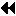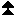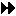## 4.12.4. Transfer of HandlesUp: Language Interoperability Next: Status Previous: Initialization

Handles are passed between Fortran and C or C++ by using an explicit C wrapper to convert Fortran handles to C handles. There is no direct access to C or C++ handles in Fortran. Handles are passed between C and C++ using overloaded C++ operators called from C++ code. There is no direct access to C++ objects from C.

The type definition MPI_Fint is provided in C/C++ for an integer of the size that matches a Fortran INTEGER; usually, MPI_Fint will be equivalent to int.

The following functions are provided in C to convert from a Fortran communicator handle (which is an integer) to a C communicator handle, and vice versa.

MPI_Comm MPI_Comm_f2c(MPI_Fint comm)

If comm is a valid Fortran handle to a communicator, then MPI_Comm_f2c returns a valid C handle to that same communicator; if comm = MPI_COMM_NULL (Fortran value), then MPI_Comm_f2c returns a null C handle; if comm is an invalid Fortran handle, then MPI_Comm_f2c returns an invalid C handle.

MPI_Fint MPI_Comm_c2f(MPI_Comm comm)

The function MPI_Comm_c2f translates a C communicator handle into a Fortran handle to the same communicator; it maps a null handle into a null handle and an invalid handle into an invalid handle.

Similar functions are provided for the other types of opaque objects.

MPI_Datatype MPI_Type_f2c(MPI_Fint datatype)

MPI_Fint MPI_Type_c2f(MPI_Datatype datatype)

MPI_Group MPI_Group_f2c(MPI_Fint group)

MPI_Fint MPI_Group_c2f(MPI_Group group)

MPI_Request MPI_Request_f2c(MPI_Fint request)

MPI_Fint MPI_Request_c2f(MPI_Request request)

MPI_File MPI_File_f2c(MPI_Fint file)

MPI_Fint MPI_File_c2f(MPI_File file)

MPI_Win MPI_Win_f2c(MPI_Fint win)

MPI_Fint MPI_Win_c2f(MPI_Win win)

MPI_Op MPI_Op_f2c(MPI_Fint op)

MPI_Fint MPI_Op_c2f(MPI_Op op)

MPI_Info MPI_Info_f2c(MPI_Fint info)

MPI_Fint MPI_Info_c2f(MPI_Info info)

Example The example below illustrates how the Fortran MPI function MPI_TYPE_COMMIT can be implemented by wrapping the C MPI function MPI_Type_commit with a C wrapper to do handle conversions. In this example a Fortran-C interface is assumed where a Fortran function is all upper case when referred to from C and arguments are passed by addresses.

```! FORTRAN PROCEDURE
SUBROUTINE MPI_TYPE_COMMIT( DATATYPE, IERR)
INTEGER DATATYPE, IERR
CALL MPI_X_TYPE_COMMIT(DATATYPE, IERR)
RETURN
END
```

```/* C wrapper */

void MPI_X_TYPE_COMMIT( MPI_Fint *f_handle, MPI_Fint *ierr)
{
MPI_Datatype datatype;

datatype = MPI_Type_f2c( *f_handle);
*ierr = (MPI_Fint)MPI_Type_commit( &datatype);
*f_handle = MPI_Type_c2f(datatype);
return;
}
```
The same approach can be used for all other MPI functions. The call to MPI_xxx_f2c (resp. MPI_xxx_c2f) can be omitted when the handle is an OUT (resp. IN) argument, rather than INOUT.

[] Rationale.

The design here provides a convenient solution for the prevalent case, where a C wrapper is used to allow Fortran code to call a C library, or C code to call a Fortran library. The use of C wrappers is much more likely than the use of Fortran wrappers, because it is much more likely that a variable of type INTEGER can be passed to C, than a C handle can be passed to Fortran.

Returning the converted value as a function value rather than through the argument list allows the generation of efficient inlined code when these functions are simple (e.g., the identity). The conversion function in the wrapper does not catch an invalid handle argument. Instead, an invalid handle is passed below to the library function, which, presumably, checks its input arguments. ( End of rationale.)

C and C++ The C++ language interface provides the functions listed below for mixed-language interoperability. The token <CLASS> is used below to indicate any valid MPI opaque handle name (e.g., Group), except where noted. For the case where the C++ class corresponding to <CLASS> has derived classes, functions are also provided for converting between the derived classes and the C MPI_<CLASS>.

The following function allows assignment from a C MPI handle to a C++ MPI handle.

MPI::<CLASS>& MPI::<CLASS>::operator=(const MPI_<CLASS>& data)

The constructor below creates a C++ MPI object from a C MPI handle. This allows the automatic promotion of a C MPI handle to a C++ MPI handle.

MPI::<CLASS>::<CLASS>(const MPI_<CLASS>& data)

Example In order for a C program to use a C++ library, the C++ library must export a C interface that provides appropriate conversions before invoking the underlying C++ library call. This example shows a C interface function that invokes a C++ library call with a C communicator; the communicator is automatically promoted to a C++ handle when the underlying C++ function is invoked.

```// C++ library function prototype
void cpp_lib_call(MPI::Comm& cpp_comm);

// Exported C function prototype
extern "C" {
void c_interface(MPI_Comm c_comm);
}

void c_interface(MPI_Comm c_comm)
{
// the MPI_Comm (c_comm) is automatically promoted to MPI::Comm
cpp_lib_call(c_comm);
}
```

The following function allows conversion from C++ objects to C MPI handles. In this case, the casting operator is overloaded to provide the functionality.

MPI::<CLASS>::operator MPI_<CLASS>() const

Example A C library routine is called from a C++ program. The C library routine is prototyped to take an MPI_Comm as an argument.

```// C function prototype
extern "C" {
void c_lib_call(MPI_Comm c_comm);
}

void cpp_function()
{
// Create a C++ communicator, and initialize it with a dup of
//   MPI::COMM_WORLD
MPI::Intracomm cpp_comm(MPI::COMM_WORLD.Dup());
c_lib_call(cpp_comm);
}
```

[] Rationale.

Providing conversion from C to C++ via constructors and from C++ to C via casting allows the compiler to make automatic conversions. Calling C from C++ becomes trivial, as does the provision of a C or Fortran interface to a C++ library. ( End of rationale.)

Note that the casting and promotion operators return new handles by value. Using these new handles as INOUT parameters will affect the internal MPI object, but will not affect the original handle from which it was cast. ( End of advice to users.)

It is important to note that all C++ objects and their corresponding C handles can be used interchangeably by an application. For example, an application can cache an attribute on MPI_COMM_WORLD and later retrieve it from MPI::COMM_WORLD.Up: Language Interoperability Next: Status Previous: Initialization